# 1.1 Repeating task n times

If you know you need to repeat something a specific number of times, use the

## 1.1.1 for loop

% for loop to sum numbers 1 to 5
sum_ = 0;
n = 5;
for iter = 1:n
sum_ = sum_ + iter;
end
fprintf('Sum of 1 to %d is %d\n', n, sum_);

# 1.2 Repeating a task when you don't know in advance when you will be done

In short, if you need a loop and a for loop won't work, use while.

## 1.2.1 while true with break

A commonly used approach is to use an infinite loop structure (while true) with a break statement to get out of the infinite loop.
% add integers until the sum is greater than 100
sum_ = 0;
n = 0;
while true
n = n + 1;
sum_ = sum_ + n;
if sum_ > 100
break
end
end
fprintf('Sum of 1 to %d is %d\n',n,sum_);

## 1.2.2 while <boolean expression>

Alternatively, the while can execute conditionally, as long as the provided boolean expression evaluates to true.
% add integers until the sum is greater than 100
sum_ = 0;
n = 0;
while sum_ < 100
n = n + 1;
sum_ = sum_ + n;
end
fprintf('Sum of 1 to %d is %d\n', n, sum_);

## 1.2.3 Validating user input

Getting correct input from the user is a perfect application of the while true with break pattern.
% get user input between 1 and 100
n = -1;
while true
n = input('Enter a number from 1 to 100: ');
if n >=1 && n <=100
break
end
end
fprintf('n = %d\n',n);

# 1.3 if, elseif, else

## 1.3.1 if

If you want to do something only if some condition is met, and there is nothing special that needs to be done if the condition is not met, then you want the if statement.
% get age, and warn user if they need adult approval
age = input('Enter your age: ');
if age < 18
end

## 1.3.2 if-else

If you want to do a task if some condition is met, and you must do a different task if the condition is not met, use the if-else pattern. Note that else does
not have a boolean expression attached to it.
% get age, and assign one of two categories
age = input('Enter your age: ');
age_category = '';
if age < 18
age_category = 'minor';
else
end
fprintf('For age = %d, category = %s\n', age, age_category);

## 1.3.3 if-elseif

If you must do one task from several possible tasks, use the if-elseif. Note that elseif does have a boolean expression attached to it.
% get age, and assign one of more than two categories
age = input('Enter your age: ');
age_category = '';
if age < 12
age_category = 'child';
elseif age < 18
age_category = 'youth';
else
end
fprintf('For age = %d, category = %s\n', age, age_category);
Also, note that the last case (the default case) used else rather than elseif. Usually, this is what your last case will do.

# 2.1 The i.i.d. Model

The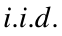model postulates that observations of the series of interest,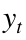, constitute a random sample. If we postulate, in addition, that the data generating process has mean μ and standard deviation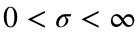, theprocess may be written as: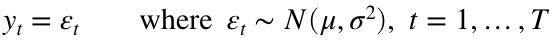This is equivalent to the linear model: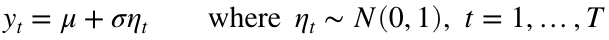Themodel is, of course, too simplistic for most empirical work, but it helps gain intuition and is a useful benchmark. Discuss whether monthly stock returns are likely to be# 2.2 Random Number Generators and Seeds

It is straightforward to generate artificial random samples of length T in MATLAB, if we know the distribution of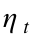including its parameters. For example, if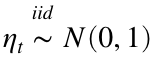, then a sample of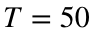observations is generated by:
randn('seed', 0);
eta = randn(50, 1);
where the first line serves to set the seed equal to an arbitrary starting value such that the code will produce the same "random" numbers each time. When generating artificial data with loops, it matters whether the seed is set inside or outside the loop. In the former case, each iteration will produce the same random draw, so make sure to set the seed outside of the loop.
Similarly, to generate a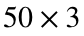matrix of mutually independent random draws from the same distribution, write:
randn('seed', 0);
eta = randn(50, 3);

# 2.3 Drawing from Pre-Specified Distributions

Often we have in mind drawing from a specific distribution for a scalar. Consider the example of a random sample of lengthfrom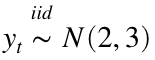. In that case, we use the rules for linear transformations of Gaussian random variables:
randn('seed', 0);
eta = randn(50, 1);
y = 2 + sqrt(3) * eta;
Of course, the last two steps can be collapsed into one line. Now consider drawing from a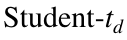distribution with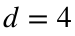degrees of freedom instead such that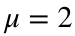and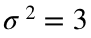:
randn('seed', 0);
eta = trnd(4, 50, 1);
% Note the ./ operation, this means element-wise division
y = 2 + sqrt(3) * (eta ./ sqrt(2));
where we made use of the fact that a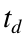-random variable has mean of 0 for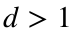and a variance offor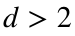. Since each element of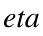has variance, we need to rescale, before adding μ and scaling by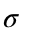. Without this transformation, the moments ofwould not meet our specifications. Figuring out the correct transformation requires familiarity with the first two moments of the underlying distribution.

# Exercises

1. Drawing from a scalar distribution: Consider drawing a random sample of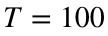observations from a rescaled and centered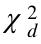-distribution withsuch that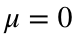and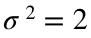. Compute the sample mean and sample variance. Then generate a second random sample of the same length from the same process. Compare the results. Repeat with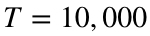. Explain the patterns in these foour sets of results.
2. Drawing from a multivariate Gaussian distribution: Generate a random sample of lengthof the trivariate random variablehaving a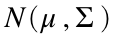distribution with:andFor this exercise you will need to find the lower triangular matrix L such that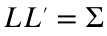and verify that the solution is correct. This may be accomplished by using the chol command in MATLAB. Keep in mind that chol generates an upper triangular matrix, so you will need to transpose the result to get L. Note that by analogy to the scalar case,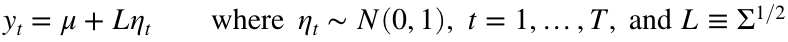Plot the data and compute the means and variances of the marginal distributions. Compute the covariance and the correlation of the second and third element of the random vector in question using the cov and std commands. Finally, construct the full correlation matrix of the random vector using the corrcoef command. Compare the results.